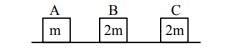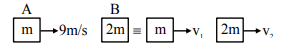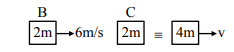Deepak Scored 45->99%ile with Bounce Back Crack Course. You can do it too!

# Three objects A, B and C are kept in a straight line on a frictionless horizontal surface.

Question:

Three objects A, B and C are kept in a straight line on a frictionless horizontal surface. The masses of A, B and C are m, 2 m and 2 m respectively. A moves towards B with a speed of 9 m/s and makes an elastic collision with it. Thereafter B makes a completely inelastic collision with C. All motions occur along same straight line. The final speed of C is :1. 6 m/s

2. 9 m/s

3. 4 m/s

4. 3 m/s

Correct Option: , 4

Solution:

Sol. Collision between A and B$\mathrm{m} \times 9=\mathrm{mv}_{1}+2 \mathrm{mv} \mathrm{v}_{2}$ (from momentum conservation)

$\mathrm{e}=1=\frac{\mathrm{v}_{2}-\mathrm{v}_{1}}{9}$

$\Rightarrow \mathrm{v}_{2}=6 \mathrm{~m} / \mathrm{sec}, \mathrm{v}_{1}=-3 \mathrm{~m} / \mathrm{sec}$

collision between $\mathrm{B}$ and $\mathrm{C}$$2 \mathrm{~m} \times 6=4 \mathrm{mv}$ (from momentum conservation)

v = 3 m/s